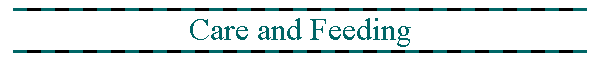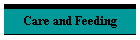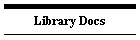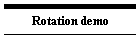# The care and feeding of Quaternions

This is one of those weird techniques where you probably won't see the need for it unless you actually try it. In 2D games featuring rotation the direction variable is usually perfectly adequate but when you are programming in 3D in Gamemaker you will probably hit a point when you need to store the orientation of an object and you will find that it is not so easy to work with 3D orientations stored as angles.

As a simple example a racing game set on a racetrack could be implemented using angles with the main angle being the direction the car is facing and the steering changing that angle but in an off-road game with slopes the vehicle may be tipped up far enough that the slope of the terrain influences the steering. In an angles-based system it is hard to allow for this but with quaternions it is easy to steer on an alternative axis. In a flight sim the player may "loop-the-loop" in which case the plane will be flying inverted. An airliner sim might get away with angles, but a stunt plane will probably need quaternions.

Quaternions are an extension of complex numbers and as such they obey some rules of algebra. They can be added, subtracted, multiplied, divided and even logs, exponents and square roots are possible. For our purposes the add and subtract operations are trivial and can be ignored.

A Unit Quaternion is a quaternion with a total magnitude of one.

Quaternions can be used to represent an axis of rotation and the degree of rotation about the axis. Multiple rotations can be combined by multiplying the quaternions and this is where it gets interesting, if an object's orientation is represented by a quaternion then the object can execute any sequence of turns, no matter how complicated, and simply multiplying the object's orientation by each rotation in turn will always yield the correct final orientation.

Orientations can also be represented as matrices, but I find Quaternions better suited to Gamemaker for the following reasons:

1. There is an easy way to apply a quaternion to an object in Gamemaker's implementation of D3D*
2. The quaternion calculation takes fewer operations than a comparable matrix operation when implemented in GML

There is an important "gotcha" in the current implementation of GM Studio in that specifying exactly 0,0,0 as a vector causes the current event to end early, probably due to a divide-by-zero. This happens specifically when ther is no rotation (unit quaternion). This could probably be fixed by testing for three zeros as the built in "epsilon" should make the comparison big enough to catch problem cases.

When programming in C those reasons for not using matrices probably will not apply, you will have more access to Direct3D and should be able to pass the matrix to it directly, and you may have access to optimised matrix ("vectorised") math libraries that will be far faster than the same number of calculations written as equations. In vectorised math the whole calculation can be handed off to a coprocessor as one action.

A Quaternion can be used to rotate a vector, this is how I implement a flying camera, I start with a vector pointing in the minus x direction and rotate it and put the result into the "set_perspective" command, I also take a z vector, rotate that and use the result as the "up" direction.

## Script library description

Since the same operations turn up again and again I've written scripts to carry out some of these operations. The script is documented in the page "Quaternions" and can be downloaded here. The script is a work-in-progress but I am confident that the parts I've written so far work consistently. I will try not to change the function of the parts I've already written, though I do intend to add functions and replace some of the longer calculations with shortened multiplied-out forms. I will put comments in the code to document it and I will try to update the description page.

Since writing it I have found a small error in the random orientation function. I believe the version on here is fixed.

## Other Scripts:

A model maker for a smooth ring donut/torus:

donut.gml

returns a model ID

The model is generated as a series of triangle_strips so the vertex count is almost half that of the same model expressed as a triangle_list form. The model has been tweaked so the texture wraps onto the torus in a similar way to the basic sphere. When the texture is applied to the torus the top and bottom edges join up. Tile-able textures work well.

## More complex than complex numbers

If we start with the idea that i is the square root of minus one, and then define j as another root of -1 where i times j is equal to minus j times i (don't question this it just works) then we can take two complex numbers (A+iB) and (C+iD) and combine them as (A+iB)+j(C+iD) or multiplied out (A+iB+jC+ijD). For convenience of notation we define k=ij giving us (A+iB+jC+kD) which is the way quaternions are often written, though in my own notes I usually abbreviate it to (A,B,C,D), and frequently refer to the four parts as q0,q1,q2,q3.

Given the rules that i.i=-1 and j.j=-1 and i.j=-j.i=k we can derive a set of rules for multiplication.

We start with determining how the complex parts i, j, k multiply:

i.j=k (given)

j.i=-i.j=-k

j.k=j.i.j=(-i.j).j=-i.j.j=-i.(-1)=i

k.j=i.j.j=i.(-1)=-i

k.i=i.j.j=-i.i.j=-(-1).j=j

i.k=i.i.j=-j

This now allows us to multiply (q0+iq1+jq2+kq3) by (r0+ir1+jr2+kr3)

=(q0(r0+ir1+jr2+kr3)+iq1(r0+ir1+jr2+kr3)+jq2(r0+ir1+jr2+kr3)+kq3(r0+ir1+jr2+kr3))

=(q0(r0+ir1+jr2+kr3)+iq1r0+iiq1r1+ijq1r2+ikq1r3+jq2r0+jiq2r1+jjq2r2+jkq2r3+kq3r0+kiq3r1+kjq3r2+kkq3r3)

=(q0(r0+ir1+jr2+kr3)+r0(iq1+jq2+kq3)-q1r1-q2r2-q3r3+i(q2r3-q3r2)+j(q3r1-q1r3)+k(q1r2-q2r1))

You do not need to understand this to use the scripts. Much of the above algebra was derived using Mathcad.

Further to the above: a Quaternion has a magnitude and a conjugate. A conjugate is a quaternion where the signs of the i,j,k parts are flipped, so (A+iB+jC+kD) becomes (A-iB-jC-kD).

Quaternions can be divided, so a multiplication can be "undone". To divide simply multiply by the inverse.

Multiplying a quaternion by it's conjugate gives the square of it's magnitude. The magnitude of a unit quaternion is always one, though in reality after your computer has processed it enough times it may start to drift due to rounding errors. Quaternions can be inverted, the inverse of a quaternion is the conjugate over the square of the magnitude.

For unit quaternions the square of the magnitude is one so the inverse is the same as the conjugate, so in our 3D environment we can undo a rotation by multiplying by the conjugate of the rotation.